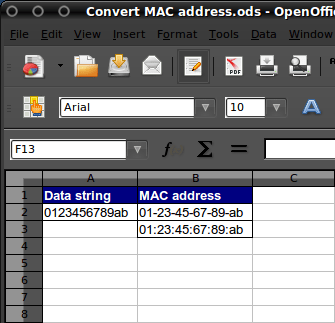Cleaning phone numbers in Excel or OO Spreadsheet

Personally I’ve been using Excel for storing phone numbers for almost twenty years now. Every now and then I don’t recognize the phone number so I need to search in my Excel sheet looking up who called me. After ten years and 500 phone numbers plus, the information stored in the Excel sheet is ‘poluted’ with hyphens and spaces. So in this article I’ll try to explain how to clean up messy telephone numbers by removing spaces and separators in Excel?

Posted on

How to split numbers and text from a string in Excel

I received an e-mail from Marc, in which he asked me whether it is posible to split numbers and text in Excel. The answer of the solution is based upon determining the first position of a number in a string, you can do that by using the following formula.

 =MIN(FIND({0;1;2;3;4;5;6;7;8;9};A1&{0;1;2;3;4;5;6;7;8;9}))

The next trick is to determine the text within a string:
A101 will become A when using the following formula

 =LEFT(A1;(MIN(FIND({0;1;2;3;4;5;6;7;8;9};A1&{0;1;2;3;4;5;6;7;8;9}))-1))

101A will become A when using the following formula

 =RIGHT(A2;(MIN(FIND({0;1;2;3;4;5;6;7;8;9};A2&{0;1;2;3;4;5;6;7;8;9}))))

To determine the numbers within a string:
A101 will become 101 when using the following formula

 =RIGHT(A1;LEN(A1)-MIN(FIND({0;1;2;3;4;5;6;7;8;9};A1&{0;1;2;3;4;5;6;7;8;9}))+1)

101A will become 101 when using the following formula

 =LEFT(A2;LEN(A2)-MIN(FIND({0;1;2;3;4;5;6;7;8;9};A2&{0;1;2;3;4;5;6;7;8;9})))

You might want to convert the text string to numbers by using the following formula.

 =VALUE()

A101 will become numeric value 101 when using the following formula

 =VALUE(RIGHT(A1;LEN(A1)-MIN(FIND({0;1;2;3;4;5;6;7;8;9};A1&{0;1;2;3;4;5;6;7;8;9}))+1))

101A will become numeric value 101 when using the following formula

 =VALUE(LEFT(A2;LEN(A2)-MIN(FIND({0;1;2;3;4;5;6;7;8;9};A2&{0;1;2;3;4;5;6;7;8;9}))))

Suggestions for improving this article are welcome, please let me know and drop me a line.

Posted on

Convert MAC address in MS Excel or OO Spreadsheet

As I’m currently working a lot with Excel, I get a lot of questions like “How do I do this?” or “How do I do that?”. Yesterday someone asked me how to convert a data string to a MAC address. The standard format for printing MAC addresses in human-friendly form is six groups of two hexadecimal digits, separated by hyphens (-) or colons (:), in transmission order, e.g. 01-23-45-67-89-ab, 01:23:45:67:89:ab. In this article I’ll give you examples of how to add, remove or change the separator for MAC addresses in MS Excel or OO Spreadsheet.

Adding a seperator (change 0123456789ab into 01-23-45-67-89-ab)=CONCATENATE(MID(A2;1;2);”-“;MID(A2;3;2);”-“;MID(A2;5;2);”-“;MID(A2;7;2);”-“;MID(A2;9;2);”-“;MID(A2;11;2)) =CONCATENATE(MID(A2;1;2);”:”;MID(A2;3;2);”:”;MID(A2;5;2);”:”;MID(A2;7;2);”:”;MID(A2;9;2);”:”;MID(A2;11;2))

An alternate solution was send in by Franco Magliaro see below:

 =MID(A2,1,2)&”-“&MID(A2,3,2)&”-“&MID(A2,5,2)&”-“&MID(A2,7,2)&”-“&MID(A2,9,2)&”-“&MID(A2,11,2) =MID(A2,1,2)&”:”&MID(A2,3,2)&”:”&MID(A2,5,2)&”:”&MID(A2,7,2)&”:”&MID(A2,9,2)&”:”&MID(A2,11,2)

Removing a separator (change 01-23-45-67-89-ab into 0123456789ab)=CONCATENATE(MID(A2;1;2);MID(A2;4;2);MID(A2;7;2);MID(A2;10;2);MID(A2;13; 2);MID(A2;16;2))

or the easy way

 =SUBSTITUTE(A2;”-“;””) =SUBSTITUTE(A2;”:”;””)

Changing a separator (change 01-23-45-67-89-ab into 01:23:45:67:89:ab)=CONCATENATE(MID(A2;1;2);”:”;MID(A2;4;2);”:”;MID(A2;7;2);”:”;MID(A2;10;2 );”:”;MID(A2;13;2);”:”;MID(A2;16;2)) =CONCATENATE(MID(A3;1;2);”-“;MID(A3;4;2);”-“;MID(A3;7;2);”-“;MID(A3;10;2 );”-“;MID(A3;13;2);”-“;MID(A3;16;2))

or the easy way 🙂

 =SUBSTITUTE(A2;”-“;”:”) =SUBSTITUTE(A2;”:”;”-“)

An alternate solution, to convert the Cisco 0123.4567.89ab MAC format to the F5 load balancer MAC format of 01:23:45:67:89:ab, was send in by Mike Wintrode. To add an extra twist, the F5 load balancer also removes the leading “0” in any pair, so for instance 00:14:0F:80:a3:01 would show as 0:14:f:80:a3:1.

So Mike created the following:

 =CONCATENATE(IF(MID(\$A2,1,1)=”0″,MID(\$A2,2,1),MID(\$A2,1,2)),”:”,IF(MID(\$A2,3,1)=”0″,MID(\$A2,4,1),MID(\$A2,3,2)),”:”,IF(MID(\$A2,6,1)=”0″,MID(\$A2,7,1),MID(\$A2,6,2)),”:”,IF(MID(\$A2,8,1)=”0″,MID(\$A2,9,1),MID(\$A2,8,2)),”:”,IF(MID(\$A2,11,1)=”0″,MID(\$A2,12,1),MID(\$A2,11,2)),”:”,IF(MID(\$A2,13,1)=”0″,MID(\$A2,14,1),MID(\$A2,13,2)))

The basic premise utilizes an “IF” statement to check for a preceding “0” and if present to return the next character, and if not to return both characters.

Adam Heath was so kind to send me an Exel template which uses most of the above formulas